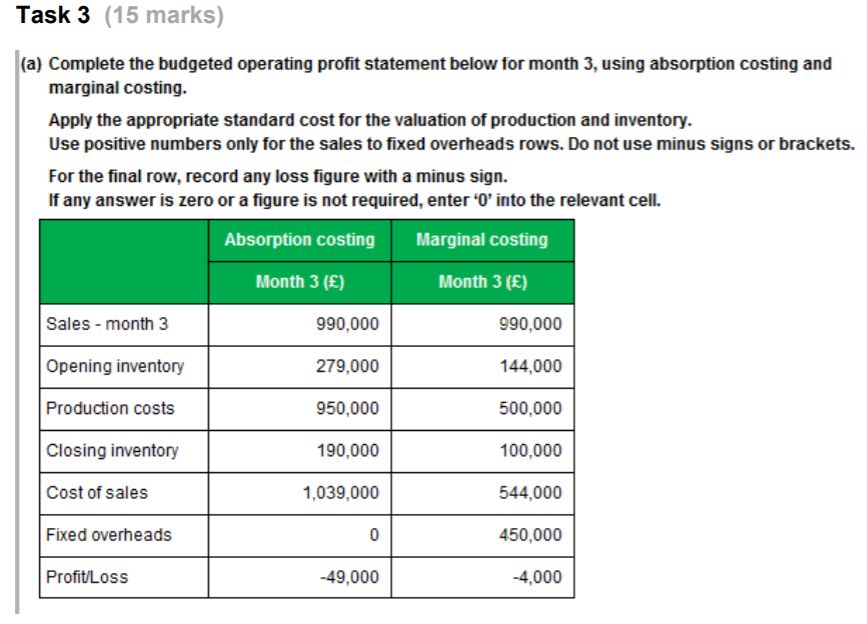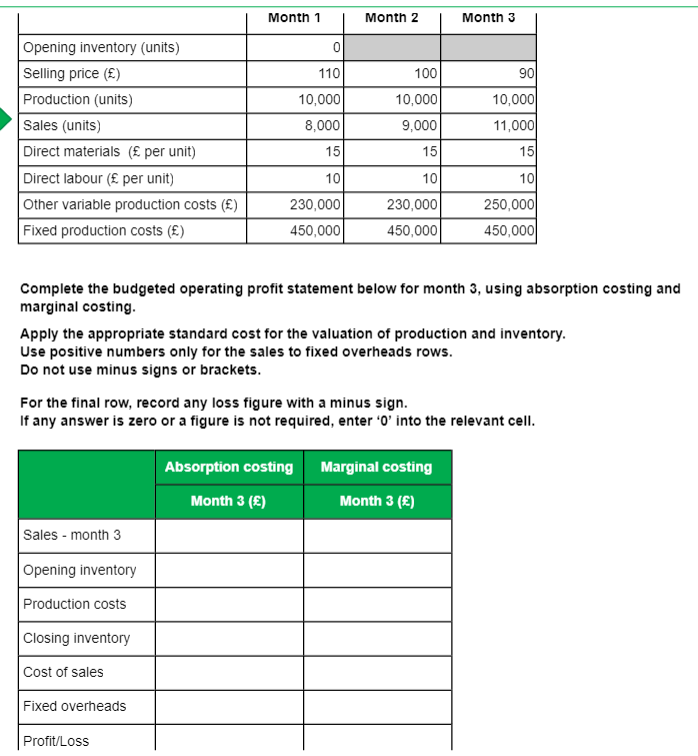# AAT Mock Test 1 Task 3.

Hi,

i'm having a mind blank and due to take my second attempt at this exam in a few weeks, please could someone explain how to get the answers please.

Thanks
Sophie• Sales units = 11,000
Selling price = 90
So, Sales = 11,000 x 90 = £990,000

Months 1 and 2:
Production units = 10,000 + 10,000 = 20,000
Sales units = 8,000 + 9,000 = 17,000
Inventory at end of Month 2 = 20,000 - 17,000 = 3,000 units

Fixed production costs = £450,000
Other variable production costs = £230,000
Production units = 10,000
Fixed production cost per unit = 450,000/10,000 = £45
Other variable production cost per unit = 230,000/10.000 = £23
Direct material per unit = £15
Direct labour per unit = £10
Variable cost per unit = 23 + 15 + 10 = £48
Total (absorption) cost per unit = 48 + 45 = £93
Marginal cost Inventory at end of month 2 (beginning of month 3) = 3,000 x 48 = £144,000
Absorption cost inventory at end of month 2 (beginning of month 3) = 3,000 x 93 = £279,000

Month 3:
Production units = 10,000
Sales units = 11,000
Inventory at end of Month 3 = 3,000 (opening) +10,000 - 11,000 = 2,000 units

Fixed production costs = as before
Other variable production costs = £250,000
Production units = 10,000
Fixed production cost per unit = £45 as before
Other variable production cost per unit = 250,000/10.000 = £25

Direct material per unit = £15
Direct labour per unit = £10
Variable cost per unit = 25 + 15 + 10 = £50
Total (absorption) cost per unit = 50 + 45 = £95
Marginal cost Inventory at end of month 3 = 2,000 x 50 = £100,000
Marginal cost production cost = 10,000 x 50 = £500,000
Absorption cost inventory at end of month 2 (beginning of month 3) = 2,000 x 95 = £190,000
Absorption cost production cost = 10,000 x 95 = £950,000

Cost of sales = opening inventory + productions costs - closing inventory
Profit = sales revenue - cost of sales
• @PeterC thank you so much for this, is there an easier way of remembering this at all?
• You're welcome

This was a fairly simple process (if you look at it a few lines at a time), there is no special technique.

The technical knowledge required, to fill in the form provided, was:

For Month 3:
opening inventory units: closing inventory for month 2 (value = units x production cost per unit)
production costs*: material + labour + variable overheads + fixed overheads (absorption)
closing inventory units: opening inventory + production units - sales units (value = units x production cost per unit)
cost of sales: opening inventory value + production costs - closing inventory value
profit/loss: sales revenue - cost of sales - fixed overheads (marginal)

* for production costs, I calculated cost per unit, then multiplied by the number of units. You can use total costs instead.

I'm sure you know all this. The secret is to just focus on one bit at a time. For each of the lines above you have to hunt though the question to find the data you need.
• @PeterC sorry to come back on this after so long but say for example the materials etc were different on months 1 and 2 which figures would you use to calculate opening etc under month 3?
• Having established that there were 3,000 items in inventory at the end of month 2, the costs I then used to value that inventory were the month 2 costs, although that isn't clearly stated in my solution. This means that it would make no difference if the month 1 costs were different to month 2.

I hope that is of some assistance.
• PeterC said:

Having established that there were 3,000 items in inventory at the end of month 2, the costs I then used to value that inventory were the month 2 costs, although that isn't clearly stated in my solution. This means that it would make no difference if the month 1 costs were different to month 2.

I hope that is of some assistance.

thanks Peter, so if just finding month 3 we use month 2 figures after working out opening and closing figures have i understood correctly?

say for example it wanted me to calculate absorption and marginal but for month 2 and 3 , would i use month one figures for opening and month 3 for closing and so on? sorry if a silly question but i have my exam tomorrow after 3 fails so trying to cover everything
• I presume you are talking about calculating the profit for the two-month period (months 2 and 3 combined). If so, you are correct. The cost of the inventory at the beginning of month 2 (end of month 1) is based on month 1 costs. The cost of inventory at the end of month 3 is based on month 3 costs

Good luck with the exam